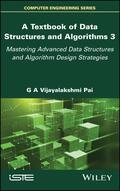John Wiley & Sons A Textbook of Data Structures and Algorithms, Volume 3Data structures and algorithms is a fundamental course in Computer Science, which enables learners a.. Product #: 978-1-78630-892-4 Regular price: \$142.06 \$142.06 In Stock

# A Textbook of Data Structures and Algorithms, Volume 3

## Mastering Advanced Data Structures and Algorithm Design Strategies1. Edition January 2023
368 Pages, Hardcover
Wiley & Sons Ltd

ISBN: 978-1-78630-892-4
John Wiley & Sons

### Price: 152,00 €

Price incl. VAT, excl. Shipping

Data structures and algorithms is a fundamental course in Computer Science, which enables learners across any discipline to develop the much-needed foundation of efficient programming, leading to better problem solving in their respective disciplines.

A Textbook of Data Structures and Algorithms is a textbook that can be used as course material in classrooms, or as self-learning material. The book targets novice learners aspiring to acquire advanced knowledge of the topic. Therefore, the content of the book has been pragmatically structured across three volumes and kept comprehensive enough to help them in their progression from novice to expert.

With this in mind, the book details concepts, techniques and applications pertaining to data structures and algorithms, independent of any programming language. It includes 181 illustrative problems and 276 review questions to reinforce a theoretical understanding and presents a suggestive list of 108 programming assignments to aid in the implementation of the methods covered.

Preface xi

Acknowledgments xvii

Chapter 13. Hash Tables 1

13.1. Introduction 1

13.2. Hash table structure 2

13.3. Hash functions 4

13.5. Chaining 13

13.6. Applications 18

13.7. Illustrative problems 23

Chapter 14. File Organizations 33

14.1. Introduction 33

14.2. Files 34

14.3. Keys 36

14.4. Basic file operations 38

14.5. Heap or pile organization 38

14.6. Sequential file organization 39

14.7. Indexed sequential file organization 41

14.8. Direct file organization 48

14.9. Illustrative problems 50

Chapter 15. k-d Trees and Treaps 61

15.1. Introduction 61

15.2. k-d trees: structure and operations 61

15.3. Treaps: structure and operations 76

15.4. Illustrative problems 83

Chapter 16. Searching 93

16.1. Introduction 93

16.2. Linear search 94

16.3. Transpose sequential search 96

16.4. Interpolation search 98

16.5. Binary search 100

16.6. Fibonacci search 104

16.7. Skip list search 108

16.8. Other search techniques 116

16.9. Illustrative problems 118

Chapter 17. Internal Sorting 131

17.1. Introduction 131

17.2. Bubble sort 132

17.3. Insertion sort 135

17.4. Selection sort 138

17.5. Merge sort 140

17.6. Shell sort 147

17.7. Quick sort 153

17.8. Heap sort 159

17.10. Counting sort 171

17.11. Bucket sort 175

17.12. Illustrative problems 179

Chapter 18. External Sorting 197

18.1. Introduction 197

18.2. External storage devices 198

18.3. Sorting with tapes: balanced merge 202

18.4. Sorting with disks: balanced merge 206

18.5. Polyphase merge sort 212

18.7. Illustrative problems 216

Chapter 19. Divide and Conquer 229

19.1. Introduction 229

19.2. Principle and abstraction 229

19.3. Finding maximum and minimum 231

19.4. Merge sort 233

19.5. Matrix multiplication 234

19.6. Illustrative problems 239

Chapter 20. Greedy Method 245

20.1. Introduction 245

20.2. Abstraction 245

20.3. Knapsack problem 246

20.4. Minimum cost spanning tree algorithms 249

20.5. Dijkstra's algorithm 251

20.6. Illustrative problems 251

Chapter 21. Dynamic Programming 261

21.1. Introduction 261

21.2. 0/1 knapsack problem 263

21.3. Traveling salesperson problem 266

21.4. All-pairs shortest path problem 269

21.5. Optimal binary search trees 272

21.6. Illustrative problems 280

Chapter 22. P and NP Class of Problems 287

22.1. Introduction 287

22.2. Deterministic and nondeterministic algorithms 289

22.3. Satisfiability problem 292

22.4. NP-complete and NP-hard problems 297

22.5. Examples of NP-hard and NP-complete problems 300

22.6. Cook's theorem 302

22.7. The unsolved problem P (?/=) NP problem 303

22.8. Illustrative problems 304

References 311

Index 313

Summaries of other volumes 317
G A Vijayalakshmi Pai, SMIEEE, is a Professor of Computer Applications at PSG College of Technology, Coimbatore, India. She has authored books and investigated research projects funded by government agencies in the disciplines of Computational Finance and Computational Intelligence.

G. A. Vijayalakshmi Pai, PSG College of Technology, Coimbatore, India